# Running accessories

51 products found
Filtres

Gender

Size

Price

€10.00 - €70.00

Couleurs

Sport

Use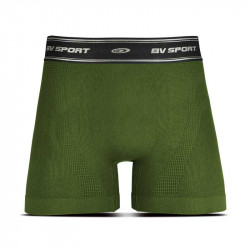• S
• M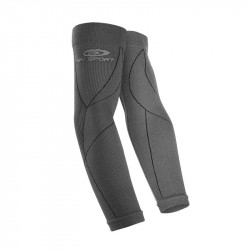• S/M
• L/XL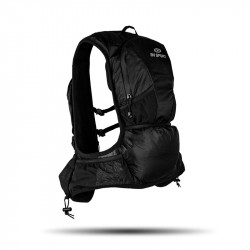• One Size• L• One Size• S
• M
• L
• XL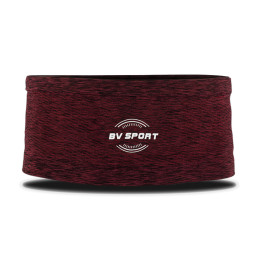• S
• M
• L• S/M
• L/XL• S
• M
• L
• XL• One Size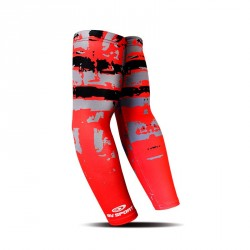• L/XL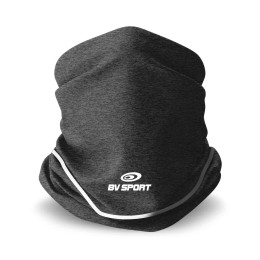• One Size• L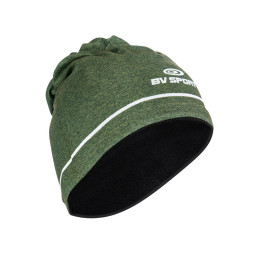• One Size• S
• M
• L• L/XL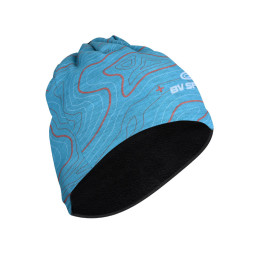• One Size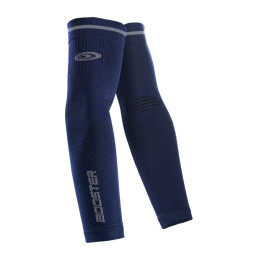• S
• M
• L• One Size• L/XL• S/M
• L/XL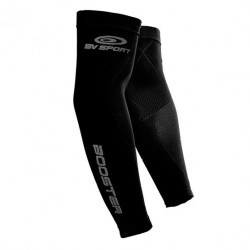• S/M
• L/XL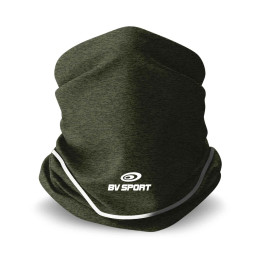• One Size• S
• M
• L
• XL• S
• M
• L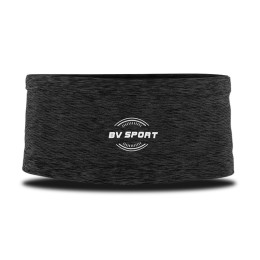• S
• M
• L• One Size• One Size• One Size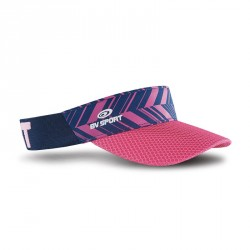• One Size• One Size• One Size• One Size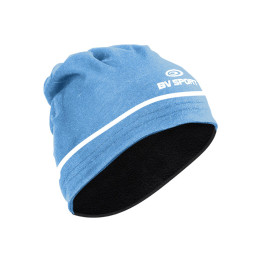• One Size• One Size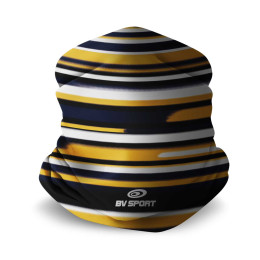• One Size